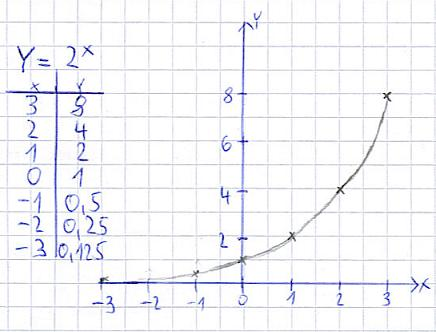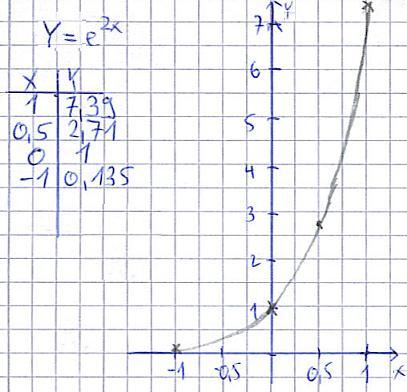# What is the graph for 4e x

## Draw the E function and the exponential function

In this section we will deal with drawing E-functions and exponential functions. We will first explain what it is all about and what Euler's number is.In the respective sections we will go into more detail about what is behind these two types. For those with little time, however, a short introduction: An exponential function is a function of the form f (x) = ax, for example f (x) = 2x or f (x) = 5x. Here a> 0 and a ≠ 1 must be. Such a function increases very quickly, especially with a capital a.

The E-function is basically described with f (x) = ex or the equation y = ex. Often, however, E-functions also include f (x) = eax + b or f (x) = k · eax + b understood, for example equations of the type y = e2x or y = e5x. The e is the so-called Euler's number, which occurs in many scientific and technical functions. In the equation we insert the rounded value e = 2.718 (or alternatively use the "e" from the calculator).

Show:

### Draw an exponential function

After we have already drawn linear functions as well as quadratic functions, we now also want to draw an exponential function. The procedure is very similar to that of the functions just mentioned. First a table of values ​​is created again and the function is drawn with it. If you are not familiar with this yet, you should first take a careful look at the two articles just mentioned. This knowledge is required for the following example. In this, y = 2x drawn. But first take a look yourself:And that's how it works:

• Create a value table
• Insert different values ​​for x and use them to calculate y
• Enter the value pairs in the coordinate system
• Connect the value pairs with a curve

That was it. As with linear and quadratic functions, different values ​​must first be inserted into the equation. Normally, different x-values ​​are used with which y is then calculated. The value pairs - for example x = 3 and y = 8 - are then entered in the coordinate system. If you have entered a few points, you draw the curve (for example with my hand as I did).

Show:

### Draw E function

Now we come to an E-function. As a reminder: The E-function is basically described with f (x) = ex or the equation y = ex. Often, however, E-functions also include f (x) = eax + b or f (x) = k · eax + b understood, for example equations of the type y = e2x or y = e5x.

The e is the so-called Euler's number, which occurs in many scientific and technical functions. In the equation we insert the rounded value e = 2.718 (or alternatively use the "e" from the calculator). And then proceed as always when drawing functions: Draw a table of values, insert different values ​​for x and thus calculate y, draw the coordinate system, enter pairs of values ​​and draw a curve.Tip: Read through our examples again thoroughly and then do the exercises. Only through extensive practice can one become confident in drawing functions. (Note: the exercises are currently being created).

Left:

### Who's Online

We have 175 guests online